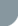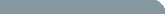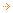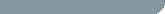Stanford University CS 226: Statistical Techniques in Robotics (Prof. Sebastian Thrun)## Midterm Exam

### Grades and statistics

Below is a summary of the grade distribution in the class after the midterm. Page 1 shows the class grades for assignments #1, #2 and the midterm. Page 2 shows the relative grades compared to class average normalized by the standard deviation.

Below is a summary of how the class did on each question on the midterm.
Midterm statistics

### Are we responsible for FastSLAM 2.0 and Sparse Extended Information Filter on the midterm?

You only need to know these algorithms at a high/conceptual level. e.g. Can you describe in 1-2 sentences what the most significant difference between FastSLAM 2.0 and 1.0 is, etc. You do not need to know the mathematical derivations of FastSLAM 2.0 or SEIF.

### Are we allowed any notes at all on the midterm?

No, no notes allowed in the exam.

## Warm-up Project

### Deliverables for the warm-up project

1. A brief summary of what you implemented in your localization algorithm--especially if you implemented something clear not covered in the textbook/lectures.
2. Demonstration of your algorithm performance including:
• Code and compile instructions.
• Output logs of most likely robot pose and sample particle locations. (You only need to propagate belief forward in time.)
• Any animations of your robot doing a fantastic job of localizing itself would be great too!

### Laser range measurement angles

The 361 measurements correspond to laser range obtained at (-90, -89.5, -89, ..., 88.5, 89, 89.5, 90) degrees respectively.

### Redundant robot pose and control signals in the log file

Since the robot pose in the log files are computed directly from control signals without taking into account laser range measurement, the two signals are redundant. i.e. If you intergrate the control signals over time, you should get the robot pose. Control signal dy should always be zero since O-bots cannot move sideways.

### Things to consider if your code is not working/running too slow...

• Make sure you get a good graphical interface first! Debugging is much easier if you have a visual representation of your localization algorithm. This applies to:
• a 2D representation of your robot in the environment,
• a cross-section profile of your laser range measurement model,
• etc.
• For those coding in matlab, I can't emphasize this enough: make sure your inner loops are very efficient.
• Although we assume the 361 laser range scans are independent variables, they really aren't. Does the range measurement at +17.5 degree really give you much more information than the range measurement at +18.0 degrees?

### Do we have to use the sample code? Can we code in Matlab?

You don't have to use the sample graphics interface. Matlab should be okay if you keep your code (i.e the inner loops) efficient.
Either way, do set up a graphical interface early on as visual debugging helps tremendously with this project.

### The data for the warm-up has been posted?

Yes. Click on "Data" on the left.

### What is the scale of the map? What are the units in the data logs?

The map scale is 1 pixel : 0.1 meter. Numbers in the logs are measured in meters.

## Written Assignment #2

### Question #4 (c): Changing the algorithm vs changing the problem

Think of this problem as asking: Suppose MCL is presented to you as a compiled library without source code. i.e. In your algorithm, you must call MCL as it is presented in Table 8.2 on page 252. Can you still produce an unbiased result?

### Question #4 (c): Does taking N to be a large number solve the bias issue?

Unfortunately, no. There is bias in the expected (however small) even for large number of N (unless N --> Infinity). The question asks for modifications that use only finite number of particles.

### Question #2: Range of valid control angles

The valid values for alpha has been changed to -89 degrees to +89 degrees. (to remove any ambiguity in the steering angle of trial #5)

### Question #3: Maximum likelihood only

You only need to compute the maximum likelihood for question #3 (exactly as stated in the question). Namely, find (v,alpha,theta') = argmax P(v,alpha,theta'|x,y,theta,x',y').

### More on bicycle motion model

Questions 1-3 are not supposed to be difficult. You're given all the freedom to approximate the bike motion. The only requirement is that your motion model should at the least be different from that of Table 5.1 in the textbook.
A key point is: the rear wheel of the bicycle is not a swivel wheel. If you push it side way, it will NOT move. Therefore, a bike should not behave the same as a garbage-can robot dragged a swivel wheel.

### Comments on bicycle motion model (questions 1 - 3)

There is no official "correct" bicycle motion model P(x'|x,u) for the purpose of grading. You are free to design any reasonble motion model provided that you can explain and demonstrate how it approximates a real bike.
EXAMPLE: Yes, you may assume the padel of the bicycle is attached either the front or rear wheel. Both will be accepted.
That being said, you should NOT model your bicycle as a point mass (a.k.a. garbage-can robot) whose motion is given in textbook Table 5.1 or a trivial extension of such robot.
The purpose of exercises 5.8.4-6 is for you to
1. write down the definition of a robot motion model
2. derive its posterior probability distribution
3. do interesting things with it! (not now but later in your warm-up project and/or research project)
You will be penalized if you reduce your motion model to that of a point mass.
EXMAPLE: Your bike should NOT travel in a straight line in the direction \$theta+alpha\$. This make it identical to a point robot centred at \$(x,y)\$ and ignores the bike frame and rear wheel. Such a model trivializes the bike motion, and you will lose points for it.
Your motion model does not need to have a closed-form solution. Both closed-form and numerical solutions are okay.

## Written Assignment #1

### 3(b) What is meant by "what is the most likely weather in hindsight?"

What are the probabilities that day N is sunny, cloudy and rainy? In other words, how do you mathematically incorporate true weather of day 1 and sensor readings from days 2, 3, ..., N+1, to produce the best prediction of the weather on day N (according to Bayes Rule). (For N = 2, 3 since there is no observation for day 5).

### Clarification on question 3

The table in question #3 gives the probability of sensor reading on day t (z_t) given the true weather on day t (x_t). Use the table from question #2 for the transition probability of weather on day t+1 (x_{t+1}) given weather on day t (x_t). Refer to figure 2.2 in the textbook or the figure from the last lecture for the relationship between states (x_t) and observations (z_t).

### Clarification on questions 3(b)

Question 3(b) asks you five separate questions:
1. 2. What is the most likely weather for day 2 (given obseration ... & ...)?
3. 4. What is the most likely weather for day 3 (given obseration ... & ...)?
5. What is the most likely weather for day 4 (given obseration ...)?
Your answer to each one of five questions should be "sunny" or "cloudy" or "rainy". Describe how you arrived at your answers.

### Clarification on questions 3(c)

Question 3(c) asks you the following two questions:
1. What is the most likely sequence of weather for days 2-4?
2. What is the probably of the above sequence of weather?
Examples of answers to the first question are "sunny-rainy-rainy", "cloudy-sunny-rainy", etc. Again, describe your formulation of the problem.

## General

### Does it matter what language we code in?

You may use any programming language most suitable. Please do include comments and compiling instructions (if applicable).

### What programming experience do I need for this course? Is MatLab an acceptable programming language for this course?

Refer to prerequisites under course overview. In general, you should be fine if you are comfortable with programming and can instruct the computer to acquire/process/display the necessary data in your course project.
For the written assignments, MatLab should be sufficient (and possibly preferrable for the shorter questions). For the warm-up project, any computation you need can be done in MatLab. Take note that MatLab is slower than compiled languages, so make sure you give yourself enough time to finish processing the data. The course project is completely open. You can choose any topic and implement in any way. Again, however, keep in mind MatLab is slower, so you may want to consider parallelizing your algorithm and/or compiling certain portions of your code.

### Is it alright to check answers with other students?

See homework policies. The written assignments are based on concepts in the textbook. We would like you to work through and understand the materials yourself.
If you do solicit help from another student, write down whom you talked to and what you learned from the discussion. In no event should you discuss the specifics of the assigned problems nor check the answers with one another.Course overviewAnnouncementsTime and locationCourse materialsScheduleInstructorsAssignmentsProjectsPoliciesDataFAQ